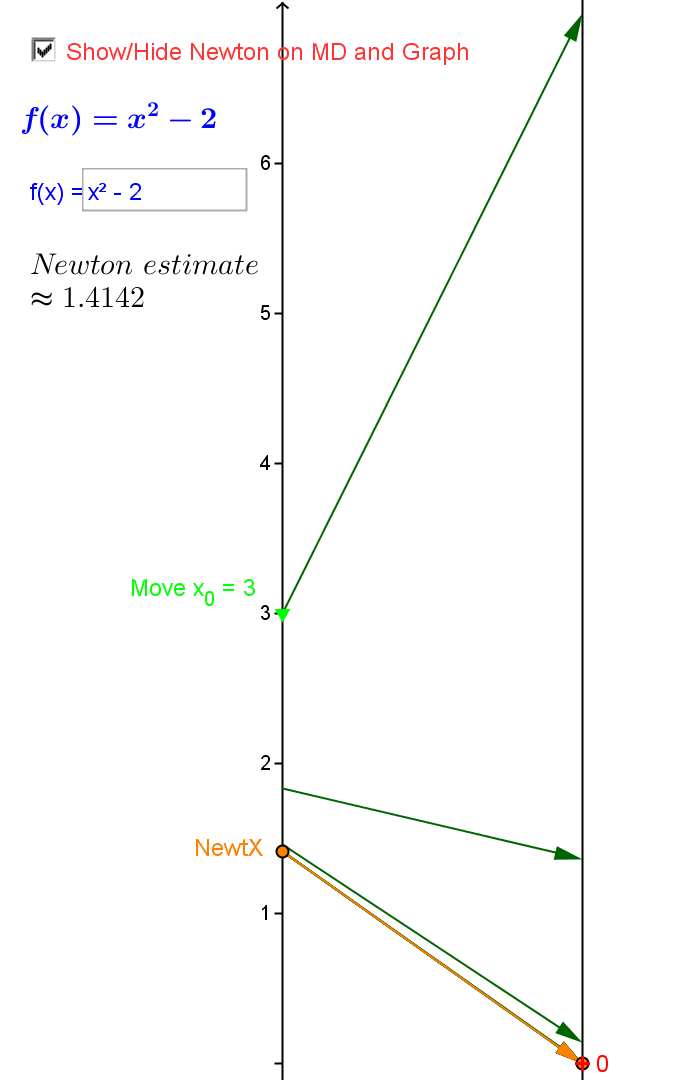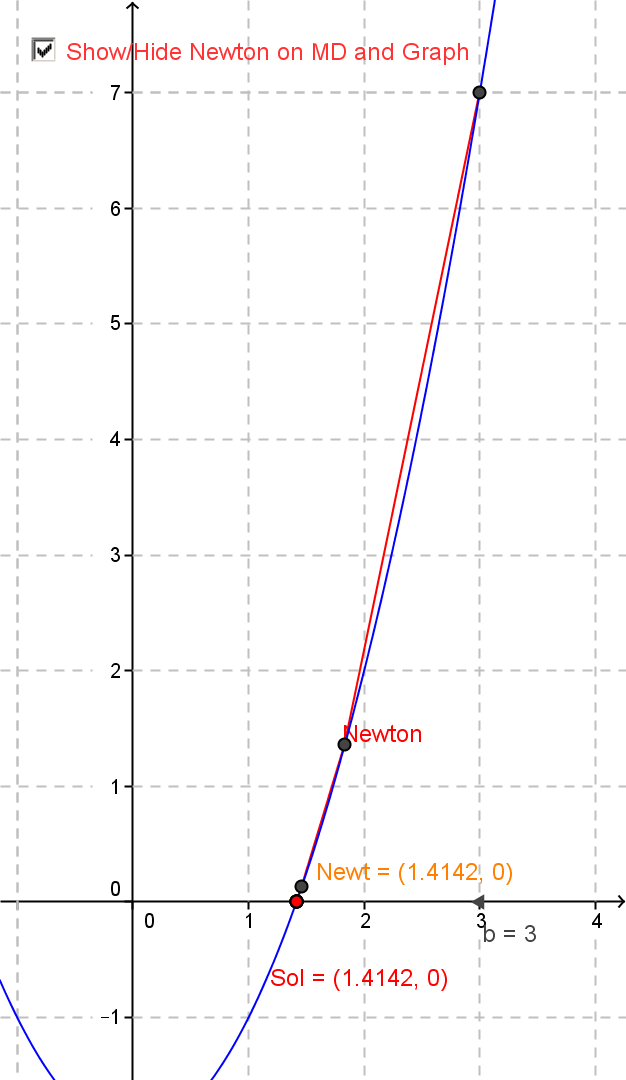Center for Recruitment and Retention of Mathematics Teachers
University of Arizona
¤
November 8, 2017

Using Mapping Diagrams to Make Sense of Functions and Calculus
Part II
Linearity, Limits, Derivatives,Differential Equations, and Integration

Martin Flashman
Professor of Mathematics
Humboldt State University

Abstract:
Participants will learn how to use mapping diagrams (MD) to make sense of functions and relate these to materials taught in calculus and in preparing for calculus.
A mapping diagram is an alternative to a Cartesian graph that visualizes a function using parallel axes. Like a table, it can present finite date, but also can be used dynamically with technology.
An overview of basic function concepts with MD’s will begin the session using worksheets and GeoGebra.
Connections of MD's to key concepts in studying calculus and preparing to study calculus will follow showing the power of MD’s to make sense of function concepts of measurement, rate, composition, and approximation related to calculus.

Background and examples will be available at Mapping Diagrams from A(lgebra) B(asics) to C(alculus) and D(ifferential) E(quation)s. A Reference and Resource for Function Visualizations Using Mapping Diagrams. http://flashman.neocities.org/MD/section-1.1VF.html

Outline for Workshop II  ¤
• Mapping Diagrams
• What is a Mapping Diagram? Review
• Brief reports on use Workshop I
• Linear Functions
• Linear functions are the key to understanding calculus.
• Nonlinear ( 1/x, trig, exp/ln) Connect to Resource
• Limits and The Derivative (visualize rate/ratio)
• An On-line Lesson on the Derivative
• Limits with Mapping Diagrams
• The Derivative As A
• Number,
• Magnification
• Rate
• Vector
• The Chain Rule: Mapping Diagrams for Composite (Linear) Functions
• An On-line Lesson on the Chain Rule
• Continuity and Solving Equations
• The Intermediate Value Theorem
• Newton's Method.
• 1st and 2nd Derivative Analysis [Time permitting]
• Differentials, Differential Equations, and Euler's Method
• Mapping Diagrams for the Differential
• Differential Equations, Euler, Mapping Diagrams
• Integration, the Fundamental Theorem(s) of Calculus, and Applications to Area.

1.Mapping Diagrams. ¤

1.2 Functions: Tables, Mapping Diagrams, and Graphs [Worksheet 1a and b, 2a and b, and 3a, b, and c.] ¤

1.3 Technology Examples  ¤

2. Linear (and Quadratic) Functions. ¤

2.3 Linear functions are the key to understanding calculus.[Worksheet II 1 and 2]
¤
Linear functions are traditionally expressed by an equation like :
$f(x)= mx + b$.
Mapping diagrams for linear functions have one simple unifying feature- the focus point, determined by the numbers
$m$ and $b$, denoted here by $[m,b]$
.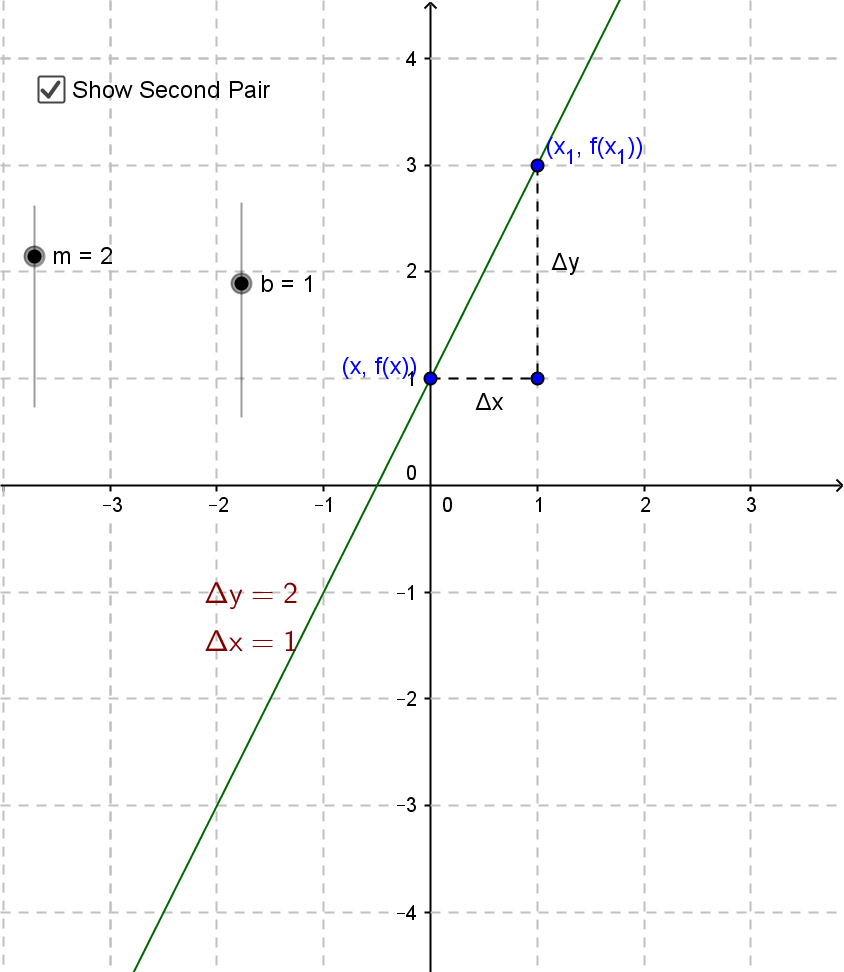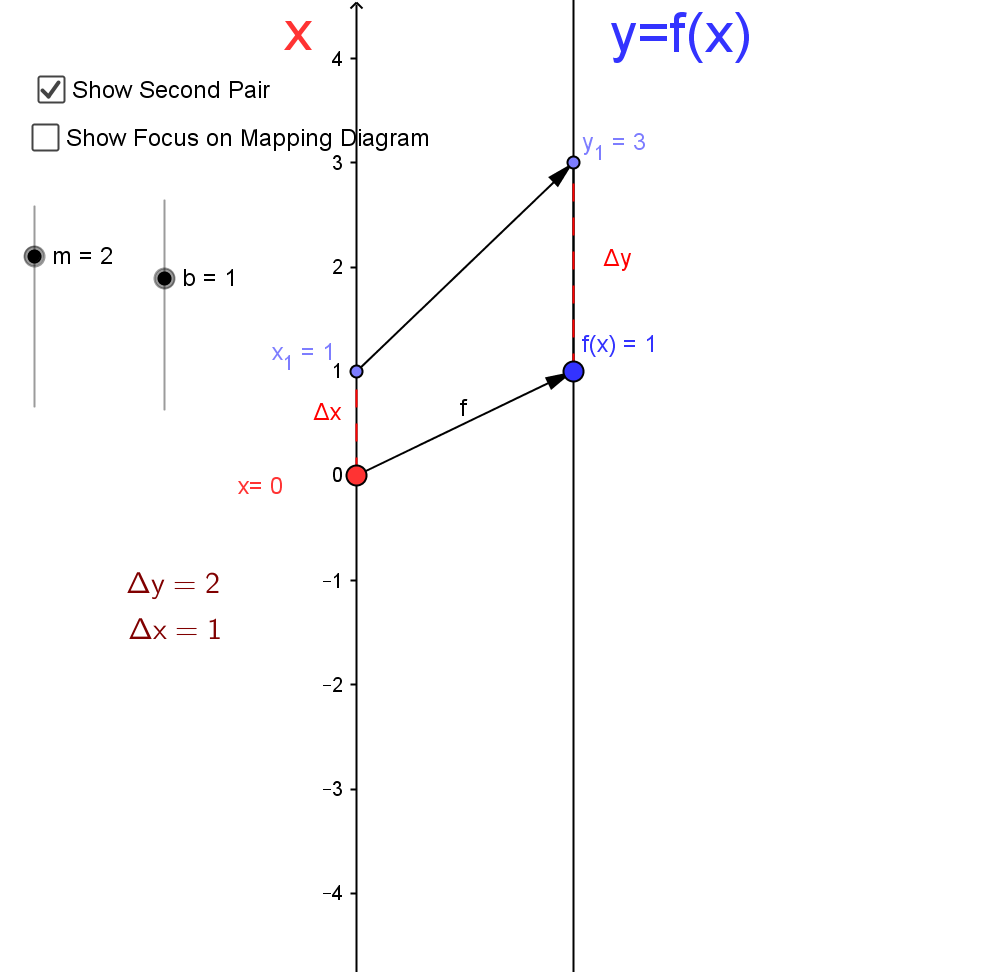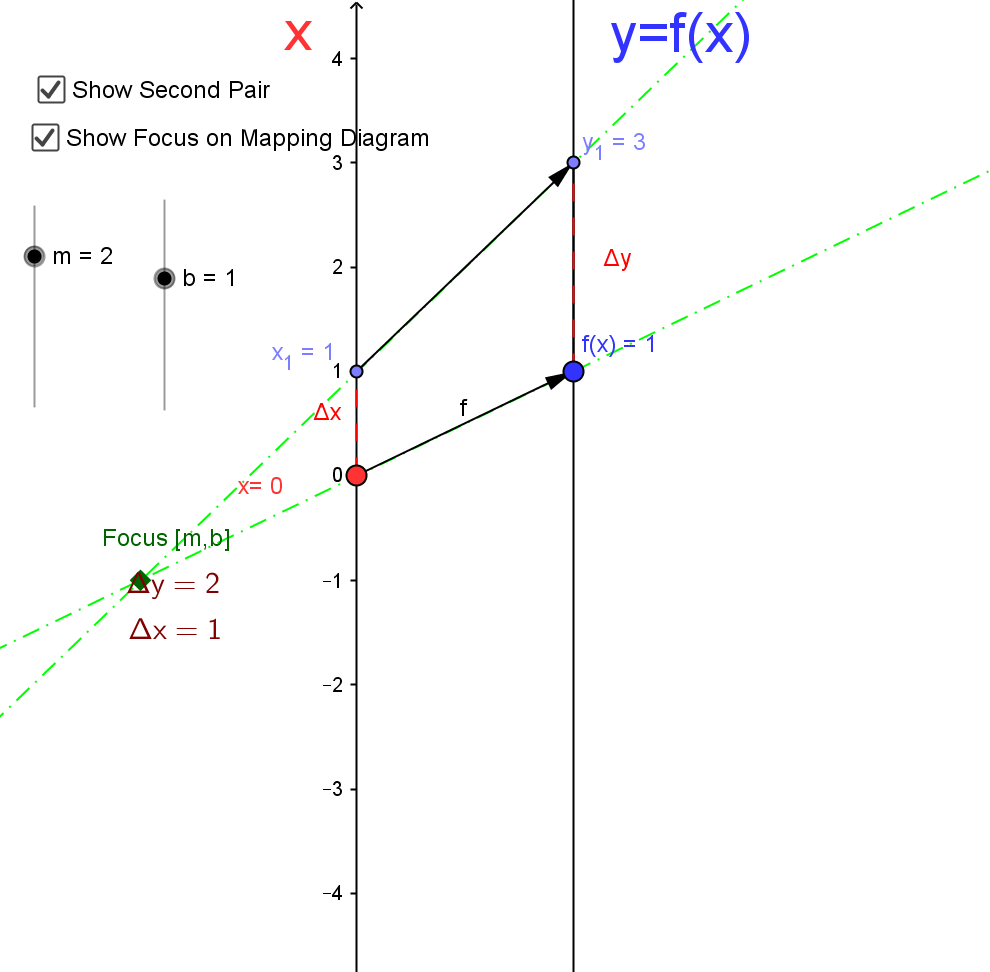Mapping Diagrams and Graphs of Linear Functions
Visualizing linear functions using mapping diagrams and graphs.
This is a Java Applet created using GeoGebra from www.geogebra.org - it looks like you don't have Java installed, please go to www.java.com
Notice how points on the graph pair with arrows and points on the mapping diagram.

2.4 Nonlinear ( 1/x, trig, exp/ln) ¤
For examples: Change functions in previous examples or connect to
Mapping Diagrams from A(lgebra) B(asics) to C(alculus) and D(ifferential) E(quation)s.

3.Limits and The Derivative ¤
Mapping Diagrams Meet Limits and The Derivative

3.0 An On-line Lesson on the Derivative
Go to Underground Mathematics (University of Cambridge):

mapping-a-derivative
With a partner start work on the Problem:
[You can use the GeoGebra applet on the linked web page.]
• What does the mapping diagram of the function $f(x)=x^2$ look like?
• What does the mapping diagram look like if it is centred on the arrow from 0
to f(0), at a scale of 1 unit per tick-mark?
• What if it is centred at some other arrow, say from 1 to f(1) or −1 to f(−1) or 32 to f(32) ?
• What does the mapping diagram look like if it is centred on the arrow from 1
to f(1), but this time zoomed in to 0.1 or 0.01
units per tick-mark?
• What if it is instead centred on some other arrow and then zoomed in?
• Can you describe what you observe?
• In what ways are the mapping diagrams of the function $f(x)=x^2$
similar to those of a linear function, and in what ways are they different?

3.1 Limits with Mapping Diagrams
[Worksheet II 3a and b.]  ¤
The traditional issue for limits (and continuity) of a function $f$ is whether
$$\lim_{x \rightarrow a}f(x) = L \ and \ \lim_{x \rightarrow a}f(x) = f(a)$$.
The definition is visualized in the following examples.
What is important even without the limit concept is the visualization of related inequalities:
Find $\delta$ so that if $a-\delta < x < a+ \delta$, then $L - \epsilon < f(x) < L+\epsilon$.
More specifically- in a linear example:  Find $\delta$ so that if $1-\delta < x < 1+ \delta$, then $1 - 0.5 < 2x-1 < 1+ 0.5$.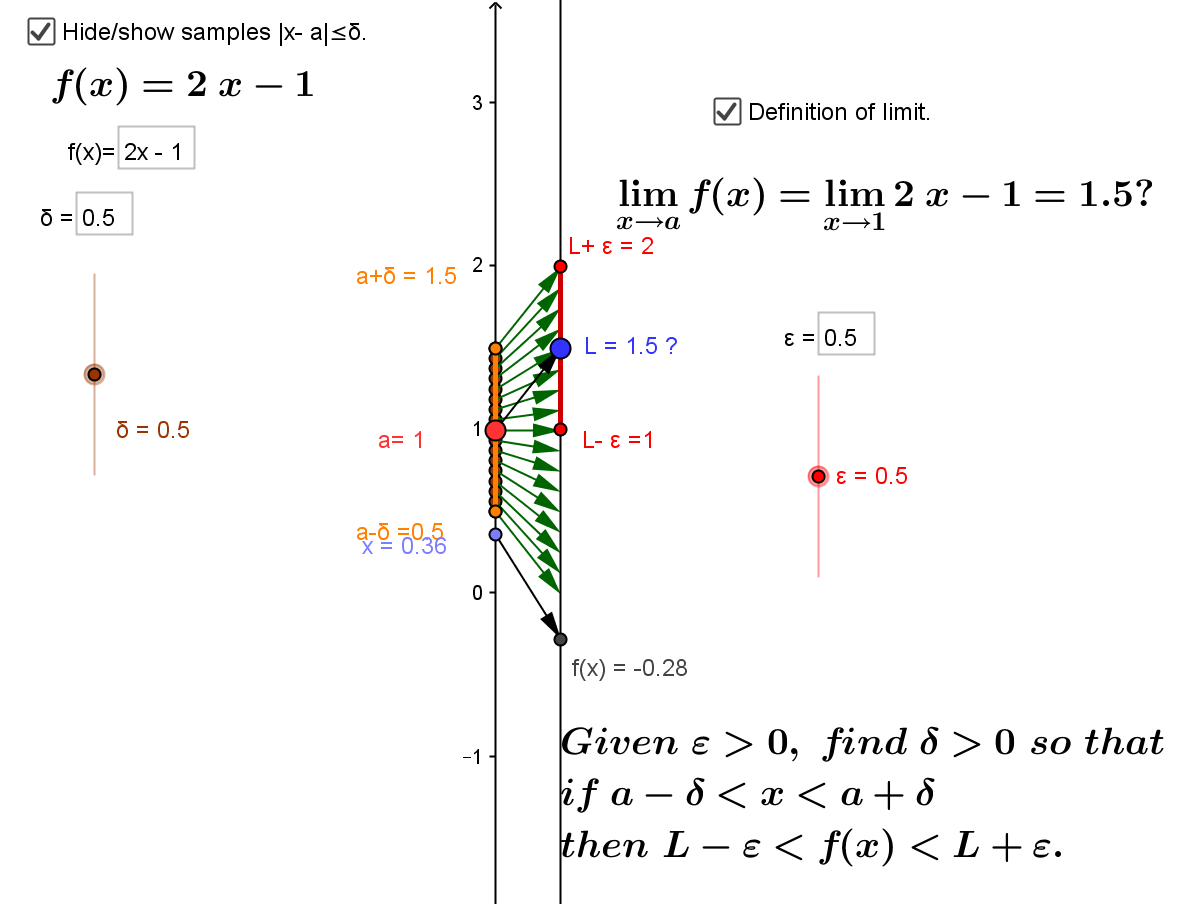Is $$\lim_{x \rightarrow a} 2x - 1 = 1.5?$$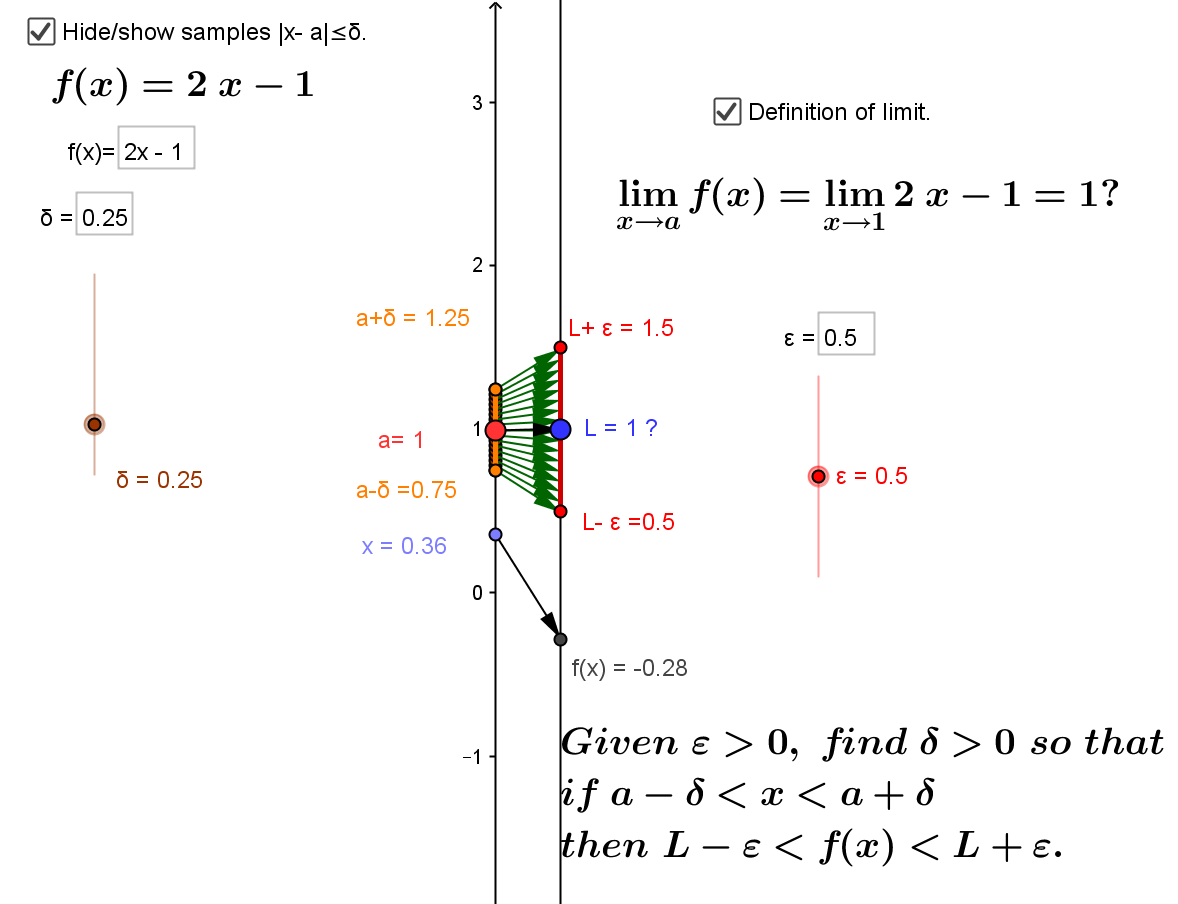Is $$\lim_{x \rightarrow a} 2x - 1 = 1 ?$$

Mapping diagrams and graphs visualize how the definition of a limit (and continuity) works for real functions.

Notice how points on the graph pair with the points and arrows on the mapping diagram.

3.2 The Derivative As A Number, Magnification, Rate Or Vector: [Worksheet II 4.]  ¤
The derivative of $f$ at $a$ is a number
, denoted $f'(a)$, defined as a limit of ratios ( average rates or slopes of lines). i.e.,
$$f '(a) = \lim_{x \to a} \frac{f(x)-f(a)}{x-a} \ or\ f'(x) = \lim_{\Delta x \to 0}\frac {f(x+\Delta x) - f(x)}{\Delta x}$$
Four Steps: I. Evaluate: $f(x+\Delta x)$ and $f(x)$
II. Subtract: $\Delta y =f(x+\Delta x) - f(x)$
III. Divide: $\frac {\Delta y}{\Delta x} =\frac {f(x+\Delta x) - f(x)}{\Delta x}$ and simplify if possible.
IV. THINK: As $\Delta x \to 0$, does $\frac {f(x+\Delta x) - f(x)}{\Delta x} \to L$ ? If so, then $L =f'(x)$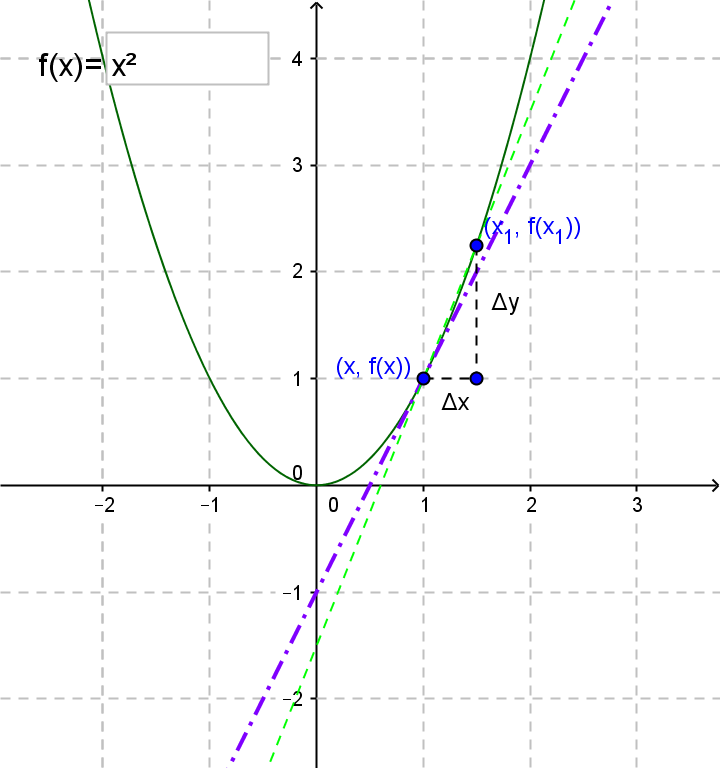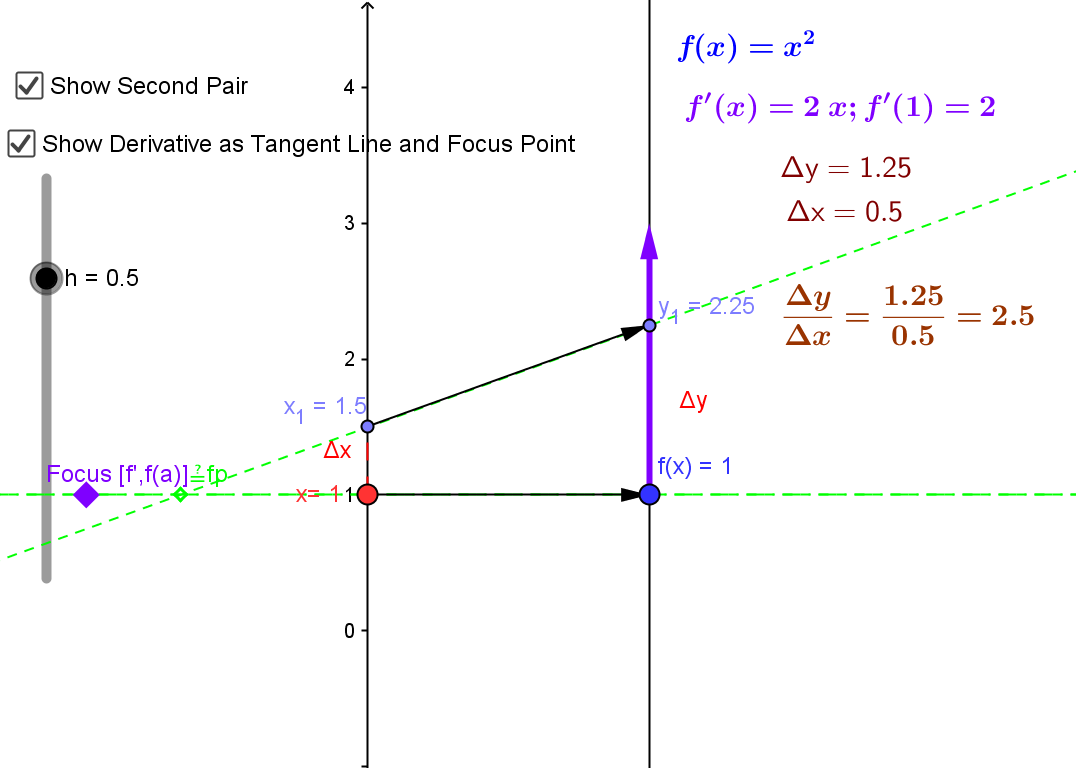The derivative can be visualized using a tangent line on a graph or a focus point and derivative "vector" on a mapping diagram.

The derivative can also be understood as the magnification factor of the best approximating linear function.¤
This is a Java Applet created using GeoGebra from www.geogebra.org - it looks like you don't have Java installed, please go to www.java.com
The derivative is visualized using focus points and derivative "vectors" on a mapping diagram.

In context of Sensible Calculus Text:  SC.I.B [Motivation] Estimating Instantaneous Velocity

3.3 The Chain Rule: Mapping Diagrams for Composite (Linear) Functions
[Worksheet II 5.] ¤
This is the fundamental concept for the chain rule.
Visualizing composite functions is a major advantage of mapping diagrams in many courses in preparation for calculus.

An On-line Lesson on the Chain Rule
Go to Underground Mathematics (University of Cambridge):
chain-mapping
With a partner start work on the Warm-Up ideas  and Problem:
[You can use the GeoGebra applet in the Interactivity tab.]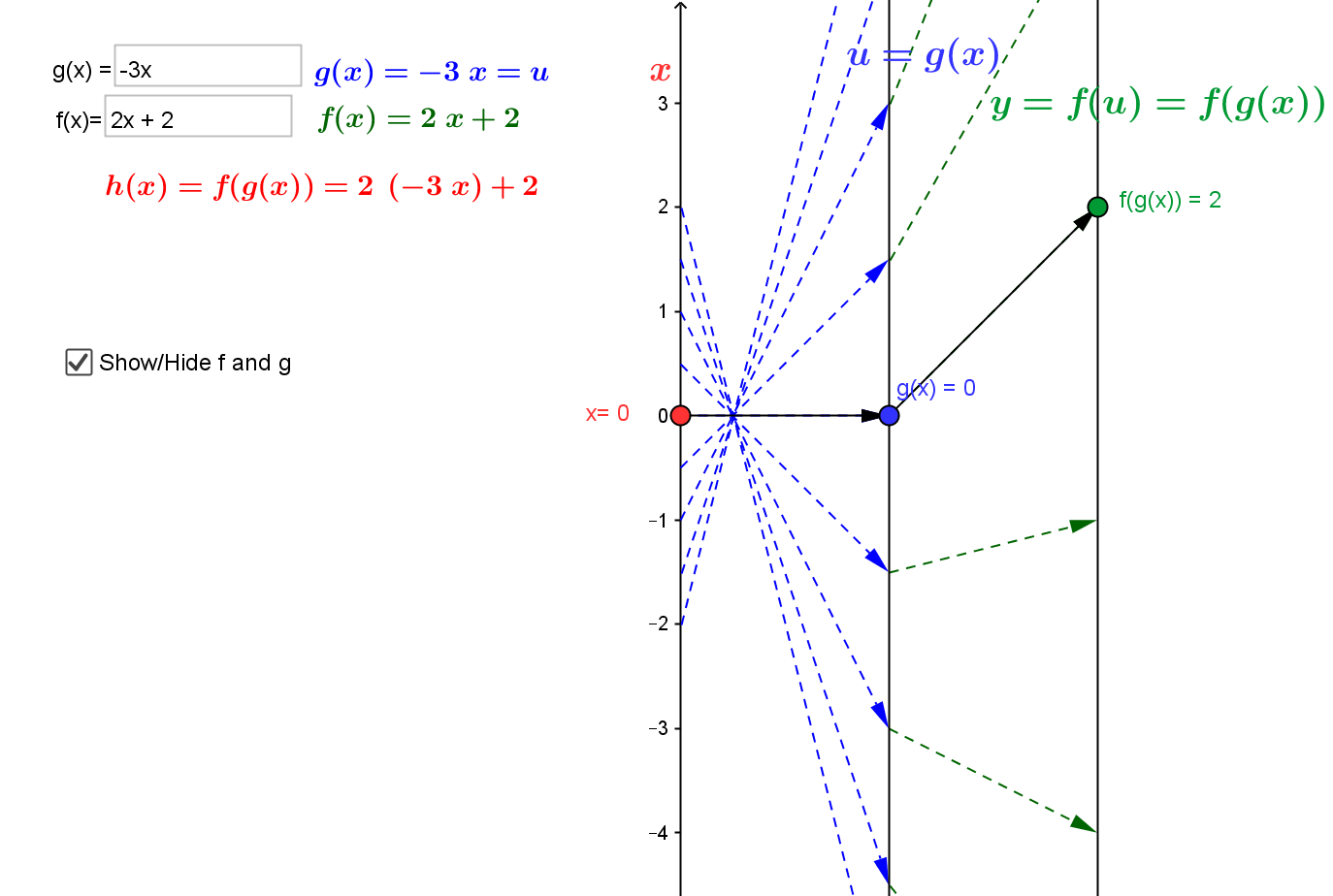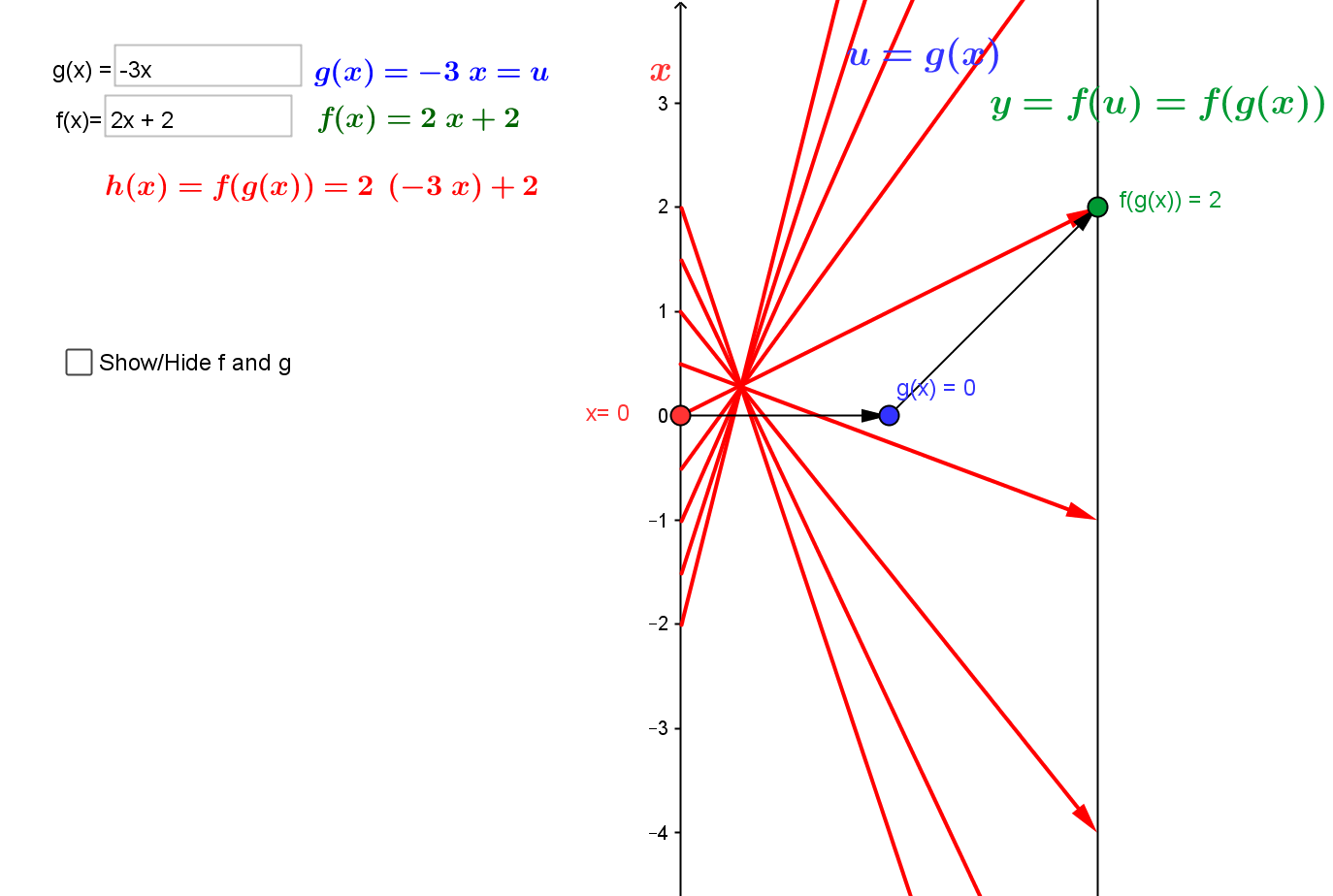Visualizing the composition of linear functions using mapping diagrams.

Composition visualized with GeoGebra.
This is a Java Applet created using GeoGebra from www.geogebra.org - it looks like you don't have Java installed, please go to www.java.com

3.4. Continuity and Solving Equations.
The Intermediate Value Theorem and Newton's Method.
¤
3.4.1. Continuity can be understood by connecting it to the Intermediate Value Theorem (IVT) and solving equations of the form $f(x) = 0$.
IVT: If $f$ is a continuous function on the interval $[a,b]$ and $f(a) \cdot f(b) \gt 0$ then there is a number $c \in (a,b)$ where $f(c) = 0$.
Mapping diagrams provide an alternative visualization for the IVT. They can also be used to visualize a proof of the result using the "bisection method."
[Worksheet II 6.]
The bisection method for solving equations is a technique that can be introduced in courses preparing for calculus- as soon as square roots are encountered.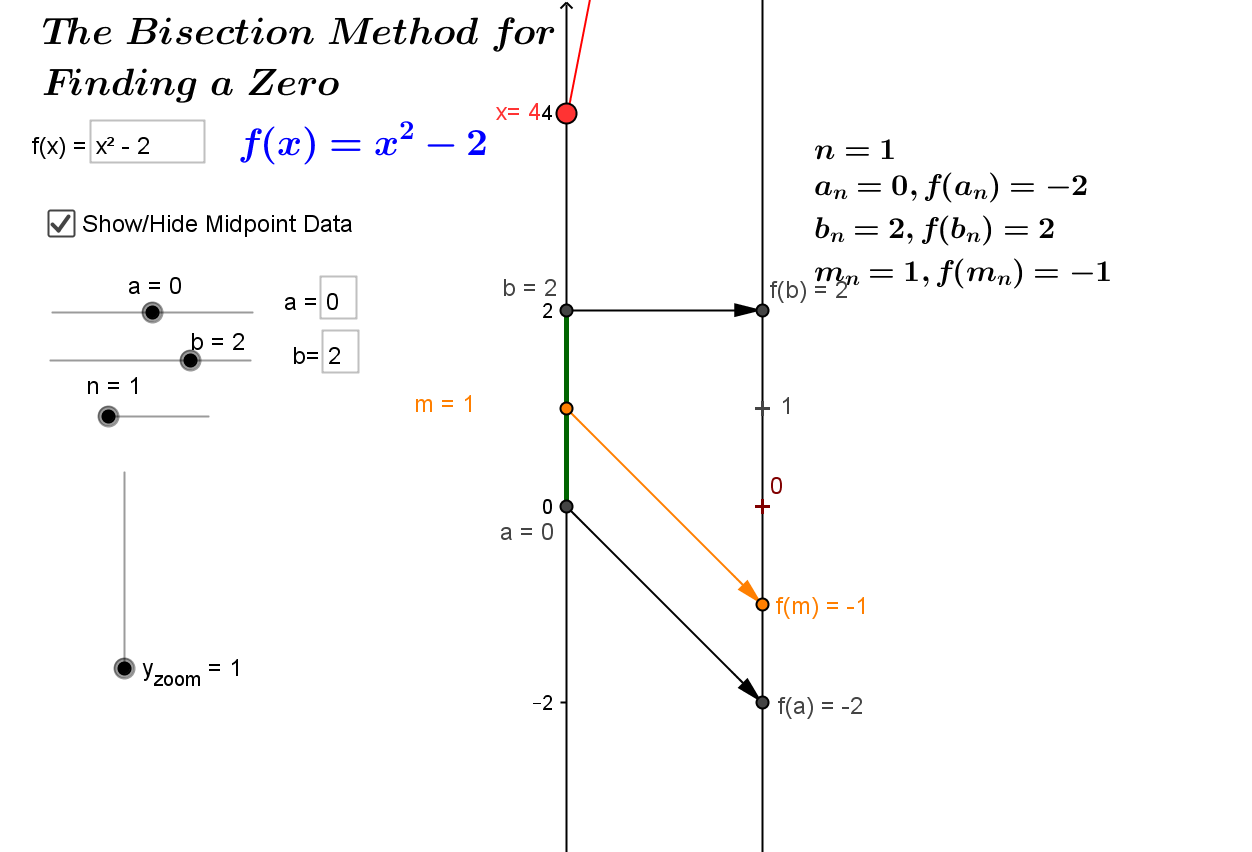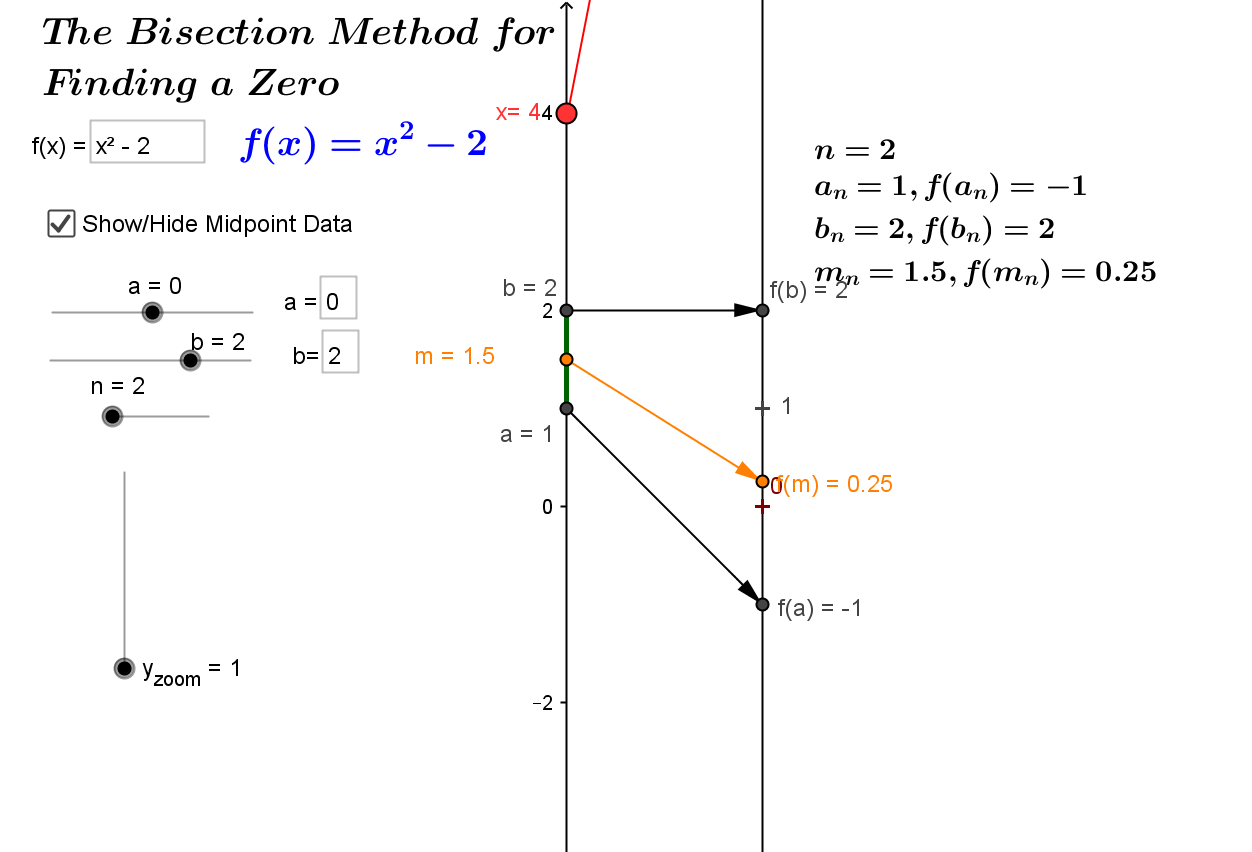Bisection and IVT vizualized with GEOGEBRA.
This is a Java Applet created using GeoGebra from www.geogebra.org - it looks like you don't have Java installed, please go to www.java.com

3.4.2 An early application of the first derivative, Newton's method for estimating roots of functions is visualized with mapping diagrams.
¤
Note: A variant of this method that uses linear interpolation can be introduced in preparation for calculus.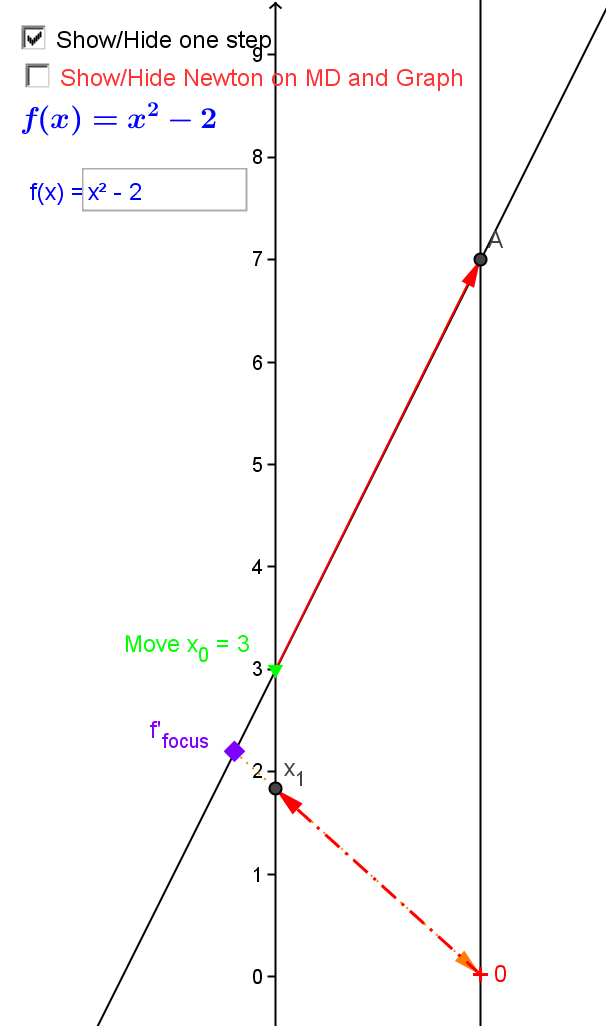The first step of Newton's method for estimating roots visualized with a mapping diagram
using the derivative focus point to find $x_1$
 $x_{n+1} =x_n - f(x_n)/f'(x_n)$ $f(x)=x^2- 2$ $f'(x)=2x$ $f(x)/f'(x) =(x^2 - 2) / (2x)$ 3.00000000000000 7.00000000000000 6.00000000000000 1.16666666666667 1.83333333333334 1.36111111111111 3.66666666666667 0.371212121212122 1.46212121212121 0.137798438934803 2.92424242424243 0.0471227822264093 1.41499842989480 0.00222055660475729 2.82999685978961 0.000784649847605263 1.41421378004720 6.15675383563997E-7 2.82842756009440 2.17674085859724E-7 1.41421356237311 4.75175454539568E-14 2.82842712474623 1.67999893079162E-14 1.41421356237310 -4.44089209850063E-16 2.82842712474619 -1.57009245868378E-16 1.41421356237310 4.44089209850063E-16 2.82842712474619 1.57009245868378E-16 1.41421356237310 -4.44089209850063E-16 2.82842712474619 -1.57009245868378E-16